# Newton's law of gravitation -- Limitations and Improvements

PainterGuy
Hi,

I was reading Wikipedia article on Newton's Law of Gravitation and I couldn't make sense of the following section.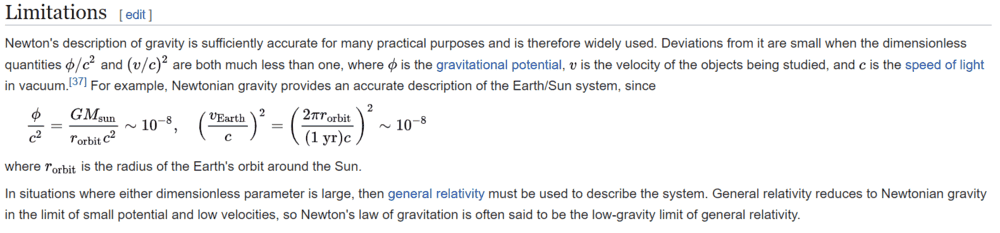Source: https://en.wikipedia.org/wiki/Newton's_law_of_universal_gravitation#Limitations

How could the improved law of gravitation be written? Like as shown below? Thank you for help in advance!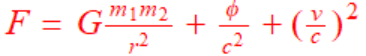Last edited by a moderator:
•Delta2

Homework Helper
Gold Member
It's not as simple as that. You may consider first reading the Wikipedia article Introduction to General Relativity here and then continue with the link to General Relativity shown in the article you quoted.

•JD_PM, Delta2, PainterGuy and 1 other person
2022 Award
Like as shown below? Thank you for help in advance!
No. Gravity isn't a force in general relativity. The fundamental equations describing the geometry of spacetime and its relationship to the matter and energy in it are the Einstein Field Equations, and the motion of free-falling particles is described by the geodesic equation. I suspect there's quite a lot of study between where you are now and being able to follow those.

That said, the Parameterised Post Newtonian model is an approximation to GR that let's you "turn on" more and more GR effects if you really need them.

Actually, approximating astronomical bodies as perfect spheres is a much worse problem than ignoring GR, at least in this solar system.

•Delta2 and PainterGuy
PainterGuy
Thank you!

If the gravity is regarded as a fictitious force, would then there be any hope to improve the Newton's law of gravitation?

I was watching the following video and from what is said there, it seems like the improved Newton's law of gravitation could be written as: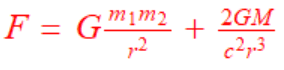The following is said around 9:33 - 10:54.
he found that his general equation for the planets reduced down to exactly Newton's equations just plus one extra term and that extra term was 2 G M over C squared R cubed so G is the big gravitational constant that tells us sort of how strong gravity generally is in the universe M is the mass of the Sun C being the speed of light and R being the distance of the planet i.e. mercury in this case from the Sun so it wasn't that Newton's equations were wrong at all it was just that that term disappears when you have either very small masses so like the things that we experience on Earth when we throw balls in the air or projectiles or very large distances between objects like with the majority of the planets in the solar system it's just that for mercury which is much closer to the Sun and so has a much smaller value of R i.e. distance from the Sun that term no longer tends to zero if you divide by a small number you get a much larger number and so that term is much more important for Mercury's orbit than it is for any other planet in the solar system so what Newton's laws really were were an approximation that just didn't happen to hold for mercury

1: https://qr.ae/pNSw5s
2: https://qr.ae/pNSwbM
3: https://physics.stackexchange.com/questions/33875/gravitation-is-not-force
4: https://physics.stackexchange.com/q...hen-how-are-forces-balanced-in-the-real-world

•Delta2
I was watching the following video and from what is said there, it seems like the improved Newton's law of gravitation could be written as:The video (at 13:05 min) also explains it's an approximation.

•PainterGuy
JD_PM
Newtonian mechanics does not yield reliable results when the speed ##v## of an object is comparable to the speed of light ##c## (i.e. ##v/c \sim 1##).

The portrayed example is the following: the Earth, with mass ##\text{M}_E##, undergoes a circular orbit around the Sun (which is not a bad approximation at all, as the eccentricity of the Earth is ##0.0167##), with mass ##\text{M}_\odot##.

Newtonian mechanics tells us that the gravitational force must equal to the centripetal one. Thus we get

\begin{equation*}
F_g = F_c \Rightarrow \frac{\text{G} \text{M}_\odot \text{M}_E}{r^2} = \frac{\text{M}_E v_E^2}{r} \Rightarrow v_E^2 = \frac{\text{G}\text{M}_\odot}{r}
\end{equation*}

Where ##r## is the distance between the Sun and the Earth (which is the definition of Astronomical Unit)

But recall that we are interested in comparing ##v## with ##c##. Thus we multiply both sides by ##1/c^2## i.e.

\begin{equation*}
\left(\frac{v_E}{c}\right)^2 = \frac{\text{G}\text{M}_\odot}{rc^2}
\end{equation*}

Plugging the following constants into it

\begin{equation*}
\text{G}=6.674 \times 10^{-11} m^3 Kg^{-1} s^{-2}
\end{equation*}

\begin{equation*}
\text{M}_\odot = 1.988 \times 10^{30} Kg
\end{equation*}

\begin{equation*}
r=1\text{AU}=1.496\times 10^{11} m
\end{equation*}

\begin{equation*}
c=2.998\times 10^{8} m/s
\end{equation*}

Indeed yields

\begin{equation*}
\left(\frac{v_E}{c}\right)^2 \sim \frac{10^{19}}{10^{27}} = 10^{-8}
\end{equation*}

Which is way off ##1##, so Newtonian Mechanics applies in this example.

Last edited:
•PainterGuy
PainterGuy
Thank you!

Could you please give the following video a look? Do you think it's good enough for a basic understanding? Thanks, in advance.

The end result given in the video is: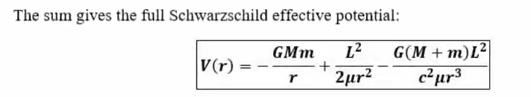JD_PM
The end result given in the video is:

View attachment 275247

Such equation is essentially correct but let us deal with its standard form

\begin{equation*}
V(r) = \underbrace{\frac 1 2 \epsilon}_{\text{const.}} -\underbrace{\frac{GM}{r}\epsilon}_{\text{Newton}} +\underbrace{\frac{L^2}{2 r^2}}_{\text{Centripetal}} -\underbrace{\frac{GML^2}{r^3}\gamma}_{\text{Einstein}}
\end{equation*}

Where ##\epsilon## determines whether the particle orbiting the mass ##M## is massive or massless: if ##\epsilon = 1## then the particle is massive, if ##\epsilon = 0## then the particle is massless (note that the former implies that the observer follows a timelike path while the latter corresponds to an observer undergoing a null trajectory. As suggested by @Ibix, you need to study further to fully understand the whole picture).

##\gamma=1## when relativistic effects are not negligible and ##\gamma=0## when Newtonian mechanics applies.

Studying the effective potential is useful to understand the types of trajectories (i.e. orbits) particles follow around massive objects.

I suggest not using software to plot the potential but sketching it yourself on a piece of paper.

In this particular problem you should study 4 cases:

1. The trajectory followed by a massless particle by means of Newtonian mechanics.
2. The trajectory followed by a massless particle by means of Einstein's relativity theory.
3. The trajectory followed by a massive particle by means of Newtonian mechanics.
4. The trajectory followed by a massive particle by means of Einstein's relativity theory.
Let us tackle 1.

Studying a massless particle (##\epsilon=0##) around a massive object under Newton's laws (##\gamma=0##) means having the potential reduced to

\begin{equation*}
V(r) = +\underbrace{\frac{L^2}{2 r^2}}_{\text{Centripetal}}
\end{equation*}

We are aimed at getting a conceptual understanding of the scenario, so we set ##L:=\sqrt{2}## (notice that the angular momentum of the system is conserved so we can always set it equal to a constant) and plot the effective potential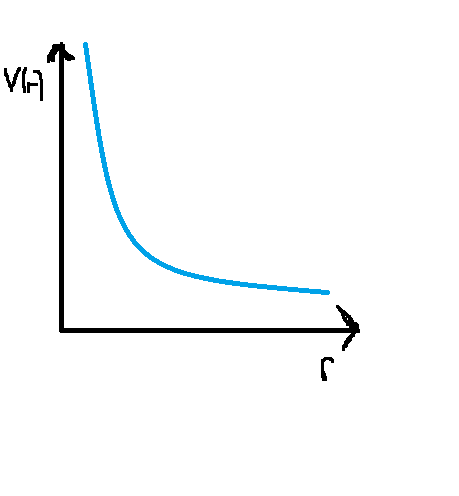What is this plot telling us about the behaviour of light when a massive object is present?

Let's say a light ray is approaching ##M## straight from ##r=+\infty##. Notice that no matter with how much energy ##E=h \nu## light carries that there will always be a turning point, as the potential is unbounded and the energy of the incoming light-ray finite (i.e. ##E<V(r)##). Thus the light ray will get closer and closer to ##M## up to hitting the turning point (i.e. the point of closest approach). Once this happens, it will go straight to ##r=+\infty##.

Conclusion: light does not get bended.

Pictorially this means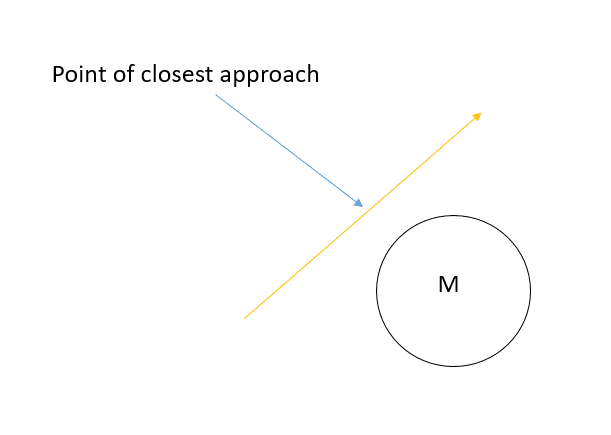If you want to prove that massless particles follow a straight line when GR effects are turned off, you will need to learn about the geodesic equation first.

I suggest you try 2., see what shape of the potential you end up getting and compare it to 1.

Hint: you should get a drastic difference.

•PainterGuy
PainterGuy
Such equation is essentially correct but let us deal with its standard form

\begin{equation*}
V(r) = \underbrace{\frac 1 2 \epsilon}_{\text{const.}} -\underbrace{\frac{GM}{r}\epsilon}_{\text{Newton}} +\underbrace{\frac{L^2}{2 r^2}}_{\text{Centripetal}} -\underbrace{\frac{GML^2}{r^3}\gamma}_{\text{Einstein}}
\end{equation*}

Thanks a lot!

Just making sure if you missed "c^2" in the denominator for the term called "Einstein"?